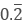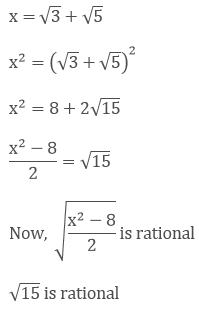Click to Chat

1800-1023-196

+91-120-4616500

CART 0

• 0

MY CART (5)

Use Coupon: CART20 and get 20% off on all online Study Material

ITEM
DETAILS
MRP
DISCOUNT
FINAL PRICE
Total Price: Rs.

There are no items in this cart.
Continue Shopping• Complete JEE Main/Advanced Course and Test Series
• OFFERED PRICE: Rs. 15,900
• View Details

```Chapter 1: Number System Exercise – 1.4

Question: 1

Define an irrational number.

Solution:

An irrational number is a real number which can be written as a decimal but not as a fraction i.e. it cannot be expressed as a ratio of integers. It cannot be expressed as terminating or repeating decimal.

Question: 2

Explain how an irrational number is differing from rational numbers?

Solution:

An irrational number is a real number which can be written as a decimal but not as a fraction i.e. it cannot be expressed as a ratio of integers. It cannot be expressed as terminating or repeating decimal.

For example, 0.10110100 is an irrational number

A rational number is a real number which can be written as a fraction and as a decimal i.e. it can be expressed as a ratio of integers. . It can be expressed as terminating or repeating decimal.

For examples,

0.10 andboth are rational numbers

Question: 3

Find, whether the following numbers are rational and irrational

(i) √7

(ii)  √4

(iii) 2 + √3

(iv) √3 + √2

(v) √3 + √5

(vi) (√2 - 2)2

(vii)  (2 - √2) (2 + √2)

(viii) (√2 + √3)2

(ix) √5 - 2

(x) √23

(xi) √225

(xii) 0.3796

(xiii) 7.478478…

(xiv) 1.101001000100001…..

Solution:

(i) √7 is not a perfect square root so it is an Irrational number.

(ii) √4 is a perfect square root so it is an rational number.

We have,

√4 can be expressed in the form of

a/b, so it is a rational number. The decimal representation of √9 is 3.0. 3 is a rational number.

(iii) 2 + √3

Here, 2 is a rational number and √3 is an irrational number

So, the sum of a rational and an irrational number is an irrational number.

(iv) √3 + √2

√3 is not a perfect square and it is an irrational number and √2 is not a perfect square and is an irrational number. The sum of an irrational number and an irrational number is an irrational number, so √3 + √2 is an irrational number.

(v) √3 + √5

√3 is not a perfect square and it is an irrational number and √5 is not a perfect square and is an irrational number. The sum of an irrational number and an irrational number is an irrational number, so √3 + √5 is an irrational number.

(vi) (√2 - 2)2

We have, (√2 - 2)2

= 2 + 4 - 4√2

= 6 + 4√2

6 is a rational number but 4√2 is an irrational number.

The sum of a rational number and an irrational number is an irrational number, so (√2 + √4)2 is an irrational number.

(vii) (2 -√2) (2 + √2)

We have,

(2 - √2) (2 + √2) = (2)2 - (√2)2 [Since, (a + b)(a - b) = a2 - b2]

4 - 2 = 2/1

Since, 2 is a rational number.

(2 - √2)(2 + √2) is a rational number.

(viii) (√2 +√3)2

We have,

(√2 + √3)2 = 2 + 2√6 + 3 = 5+√6   [Since, (a + b)2 = a2 + 2ab + b2

The sum of a rational number and an irrational number is an irrational number, so (√2 + √3)2 is an irrational number.

(ix) √5 - 2

The difference of an irrational number and a rational number is an irrational number. (√5 - 2) is an irrational number.

(x) √23

√23 = 4.795831352331….

As decimal expansion of this number is non-terminating, non-recurring so it is an irrational number.

(xi) √225

√225 = 15 = 15/1

√225 is rational number as it can be represented in p/q form.

(xii) 0.3796

0.3796, as decimal expansion of this number is terminating, so it is a rational number.

(xiii) 7.478478……

7.478478 = 7.478, as decimal expansion of this number is non-terminating recurring so it is a rational number.

(xiv) 1.101001000100001……

1.101001000100001……, as decimal expansion of this number is non-terminating, non-recurring so it is an irrational number

Question: 4

Identify the following as irrational numbers. Give the decimal representation of rational numbers:

(i) √4

(ii) 3 × √18

(iii) √1.44

(iv) √(9/27)

(v) - √64

(vi) √100

Solution:

(i) We have,

√4 can be written in the form of

p/q. So, it is a rational number. Its decimal representation is 2.0

(ii). We have,

3 × √18

= 3 × √2 × 3 × 3

= 9×√2

Since, the product of a ratios and an irrational is an irrational number. 9 ×√2 is an irrational.

3 ×√18 is an irrational number.

(iii) We have,

√1.44

= √(144/100)

= 12/10

= 1.2

Every terminating decimal is a rational number, so 1.2 is a rational number.

Its decimal representation is 1.2.

(iv) √(9/27)

We have,

√(9/27)

=3/√27

= 1/√3

Quotient of a rational and an irrational number is irrational numbers so

1/√3 is an irrational number.

√(9/27) is an irrational number.

(v) We have,

-√64

= - 8

= - (8/1)

= - (8/1) can be expressed in the form of a/b,

so - √64 is a rational number.

Its decimal representation is - 8.0.

(vi) We have,

√100

= 10  can be expressed in the form of a/b,

So √100 is a rational number

Its decimal representation is 10.0.

Question: 5

In the following equations, find which variables x, y and z etc. represent rational or irrational numbers:

(i) x2 = 5

(ii) y2 = 9

(iii) z2 = 0.04

(iv) u2 = 174

(v) v2 = 3

(vi) w2 = 27

(vii) t2 = 0.4

Solution:

(i) We have,

x2 = 5

Taking square root on both the sides, we get

X = √5

√5 is not a perfect square root, so it is an irrational number.

(ii) We have,

= y2 = 9

= 3

= 3/1 can be expressed in the form of a/b, so it a rational number.

(iii) We have,

z2 = 0.04

Taking square root on the both sides, we get

z = 0.2

2/10 can be expressed in the form of a/b, so it is a rational number.

(iv) We have,

u2 = 17/4

Taking square root on both sides, we get,

u = √(17/4)

u = √17/2

Quotient of an irrational and a rational number is irrational, so u is an Irrational number.

(v) We have,

v2 = 3

Taking square root on both sides, we get,

v = √3

√3 is not a perfect square root, so v is irrational number.

(vi) We have,

w2 = 27

Taking square root on both the sides, we get,

w = 3√3

Product of a irrational and an irrational is an irrational number. So w is an irrational number.

(vii) We have,

t2 = 0.4

Taking square root on both sides, we get,

t = √(4/10)

t = 2/√10

Since, quotient of a rational and an Irrational number is irrational number. t2 = 0.4 is an irrational number.

Question: 6

Give an example of each, of two irrational numbers whose:

(i) Difference in a rational number.

(ii) Difference in an irrational number.

(iii) Sum in a rational number.

(iv) Sum is an irrational number.

(v) Product in a rational number.

(vi) Product in an irrational number.

(vii) Quotient in a rational number.

(viii) Quotient in an irrational number.

Solution:

(i) √2 is an irrational number.

Now, √2 -√2 = 0.

0 is the rational number.

(ii) Let two irrational numbers are 3√2 and √2.

3√2 - √2 = 2√2

5√6 is the rational number.

(iii) √11 is an irrational number.

Now, √11 + (-√11) = 0.

0 is the rational number.

(iv) Let two irrational numbers are 4√6 and √6

4√6 + √6

5√6 is the rational number.

(iv) Let two Irrational numbers are 7√5 and √5

Now, 7√5 × √5

= 7 × 5

= 35 is the rational number.

(v) Let two irrational numbers are √8 and √8.

Now, √8 × √8

8 is the rational number.

(vi) Let two irrational numbers are 4√6 and √6

Now, (4√6)/√6

= 4 is the rational number

(vii) Let two irrational numbers are 3√7 and √7

Now, 3 is the rational number.

(viii) Let two irrational numbers are √8 and √2

Now √2 is an rational number.

Question: 7

Give two rational numbers lying between 0.232332333233332 and 0.212112111211112.

Solution:

Let a = 0.212112111211112

And, b = 0.232332333233332...

Clearly, a < b because in the second decimal place a has digit 1 and b has digit 3 If we consider rational numbers in which the second decimal place has the digit 2, then they will lie between a and b.

Let. x = 0.22

y = 0.22112211... Then a < x < y < b

Hence, x, and y are required rational numbers.

Question: 8

Give two rational numbers lying between 0.515115111511115 and 0. 5353353335

Solution:

Let, a = 0.515115111511115...

And, b = 0.5353353335..

We observe that in the second decimal place a has digit 1 and b has digit 3, therefore, a < b.

So If we consider rational numbers

x = 0.52

y = 0.52062062...

We find that,

a < x < y < b

Hence x and y are required rational numbers.

Question: 9

Find one irrational number between 0.2101 and 0.2222 ... =Solution:

Let, a = 0.2101 and,

b = 0.2222...

We observe that in the second decimal place a has digit 1 and b has digit 2, therefore a < b in the third decimal place a has digit 0.

So, if we consider irrational numbers

x = 0.211011001100011....

We find that a < x < b

Hence x is required irrational number.

Question: 10

Find a rational number and also an irrational number lying between the numbers 0.3030030003... and 0.3010010001...

Solution:

Let,

a = 0.3010010001 and,

b = 0.3030030003...

We observe that in the third decimal place a has digit 1 and b has digit

3, therefore a < b in the third decimal place a has digit 1. So, if we

consider rational and irrational numbers

x = 0.302

y = 0.302002000200002.....

We find that a < x < b and, a < y < b.

Hence, x and y are required rational and irrational numbers respectively.

Question: 11

Find two irrational numbers between 0.5 and 0.55.

Solution:

Let a = 0.5 = 0.50 and b = 0.55

We observe that in the second decimal place a has digit 0 and b has digit

5, therefore a < 0 so, if we consider irrational numbers

x = 0.51051005100051...

y = 0.530535305353530...

We find that a < x < y < b

Hence x and y are required irrational numbers.

Question: 12

Find two irrational numbers lying between 0.1 and 0.12.

Solution:

Let a = 0.1 = 0.10

And b = 0.12

We observe that In the second decimal place a has digit 0 and b has digit 2.

Therefore, a < b.

So, if we consider irrational numbers

x = 0.1101101100011... y = 0.111011110111110... We find that a < x < y < 0

Hence, x and y are required irrational numbers.

Question: 13

Prove that √3 + √5 is an irrational number.

Solution:

If possible, let √3 + √5 be a rational number equal to x.

Then,Thus, we arrive at a contradiction.

Hence, √3 + √5 is an irrational number.
```### Course Features

• 728 Video Lectures
• Revision Notes
• Previous Year Papers
• Mind Map
• Study Planner
• NCERT Solutions
• Discussion Forum
• Test paper with Video Solution